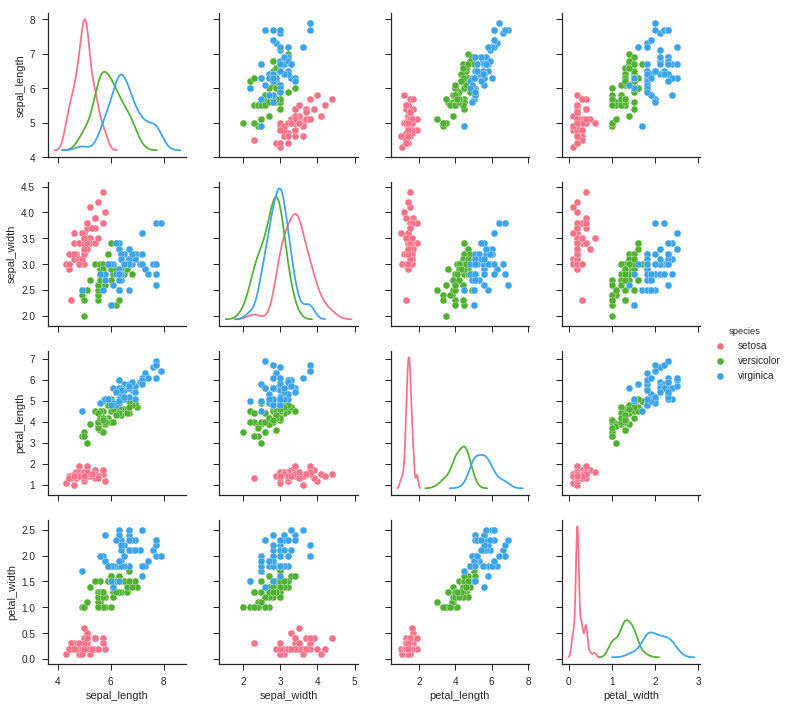# Seaborn - Working On Visualising Pairwise Relationship¶

Datasets under real-time study contain many variables. In such cases, the relation between each and every variable should be analyzed. Plotting Bivariate Distribution for (n,2) combinations will be a very complex and time taking process.

To plot multiple pairwise bivariate distributions in a dataset, you can use the pairplot() function. This shows the relationship for (n,2) combination of a variable in a DataFrame as a matrix of plots and the diagonal plots are the univariate plots.

Axes In this section, we will learn what are Axes, their usage, parameters, and so on.

### Usage¶

seaborn.pairplot(data,…)

### Parameters¶

Following table lists down the parameters for Axes −

Sr.No. Parameter & Description

• Data

Dataframe

• Hue

Variable in data to map plot aspects to different colors.

• Palette

Set of colors for mapping the hue variable

• Kind

Kind of plot for the non-identity relationships. {‘scatter’, ‘reg’}

• Diag_kind

Kind of plot for the diagonal subplots. {‘hist’, ‘kde’}

Except data, all other parameters are optional. There are few other parameters which pairplot can accept. The above mentioned are often used params.

In :
import pandas as pd
import seaborn as sb
from matplotlib import pyplot as pltDolly Solanki